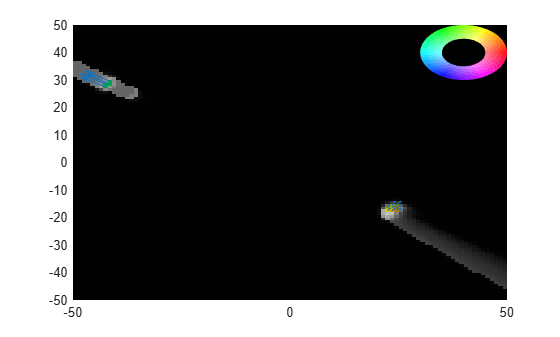# show

Visualize dynamic evidential grid map

## Syntax

``show(map)``
``show(map,Name,Value)``

## Description

````show(map)` plots the dynamic occupancy grid map in local coordinates. The static cells are shown using grayscale images, in which the grayness represents the occupancy probability of the cell. The dynamic cells are shown using HSV (hue, saturation, and value) values on an RGB colormap: Hue — The orientation angle of the velocity vector divided by 360. As hue increases from 0 to 1, the color changes in the order of red to orange, yellow, green, cyan, blue, magenta, and back to red. Saturation — The Mahalanobis distance (d) between the velocity distribution of the grid cell and the zero velocity. A cell with d > 4 is drawn with full saturation.Value — The occupancy probability of the cell. ```

example

````show(map,Name,Value)` specifies options using one or more name-value pair arguments. Enclose each Name in quotes. For example, `show(myMap,'PlotVelocity',true)` plots the dynamic map for `myMap` with velocity plotting enabled.```

## Examples

collapse all

Load a `dynamicEvidentialGridMap` object from a data file.

```load gridMapData.mat map```
```map = dynamicEvidentialGridMap with properties: NumStateVariables: 4 MotionModel: 'constant-velocity' GridLength: 100 GridWidth: 100 GridResolution: 1 GridOriginInLocal: [-50 -50] ```

Show the grid map.

```figure show(map)```Show the grid map with velocity plotted and color inverted.

```figure show(map,'PlotVelocity',true,'InvertColors',true)```## Input Arguments

collapse all

Dynamic evidential grid map, specified as a `dynamicEvidentialGridMap` object.

### Name-Value Arguments

Specify optional pairs of arguments as `Name1=Value1,...,NameN=ValueN`, where `Name` is the argument name and `Value` is the corresponding value. Name-value arguments must appear after other arguments, but the order of the pairs does not matter.

Before R2021a, use commas to separate each name and value, and enclose `Name` in quotes.

Example: `showDynamicMap(myTracker,'PlotVelocity',false)` plots the dynamic map for `myTracker` with velocity plotting enabled.

Enable velocity plotting, specified as `true` or `false`. When specified as `true`, the velocity vector for each dynamic cell is plotted at the center of the grid cell. The length of the plotted vector represents the magnitude of the velocity.

Parent axes on which to plot the map, specified as an `axes` handle.

Enable updating from previous map, specified as `true` or `false`. When specified as `true`, the function plots the map via a lightweight update to the previous map in the figure. When specified as `false`, the function plots a new map on the figure every time.

Enable inverted colors on the map, specified as `true` or `false`. When specified as `false`, the function plots empty space in white and occupied space in black. When specified as `ture`, the function plots empty space in black and occupied space in white.

## Version History

Introduced in R2021a